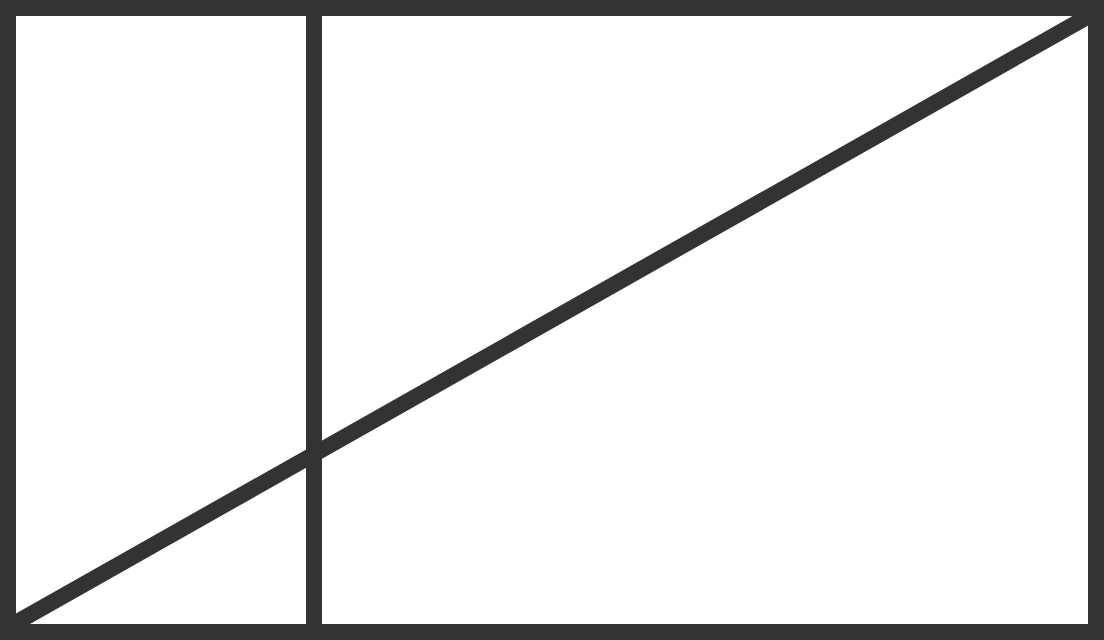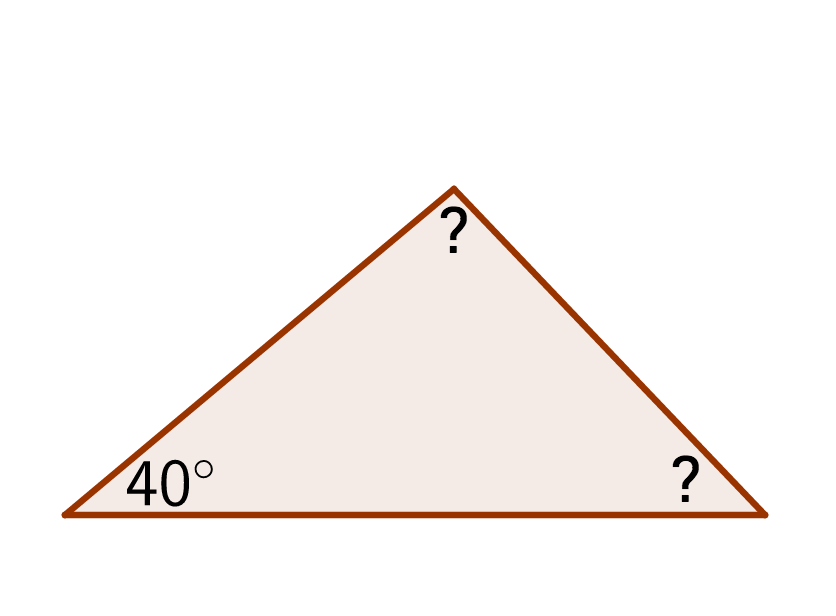Geometry

# Classification of Triangles Warmup

True or False?

A right triangle can be isosceles.Above, a line cuts across two adjacent rectangles.

How many right triangles can you find in this diagram?

Two sides of an isosceles triangle measure 3 and 5. What could be the length of the third side?Eli needs to draw an obtuse triangle and begins by creating a $$40^\circ$$ angle. What could be the measure of the next angle he draws? (In an obtuse triangle, one angle measures more than $$90^\circ.$$

What type of triangle has both an $$89^\circ$$ angle and a $$1^\circ$$ angle?

×

Problem Loading...

Note Loading...

Set Loading...